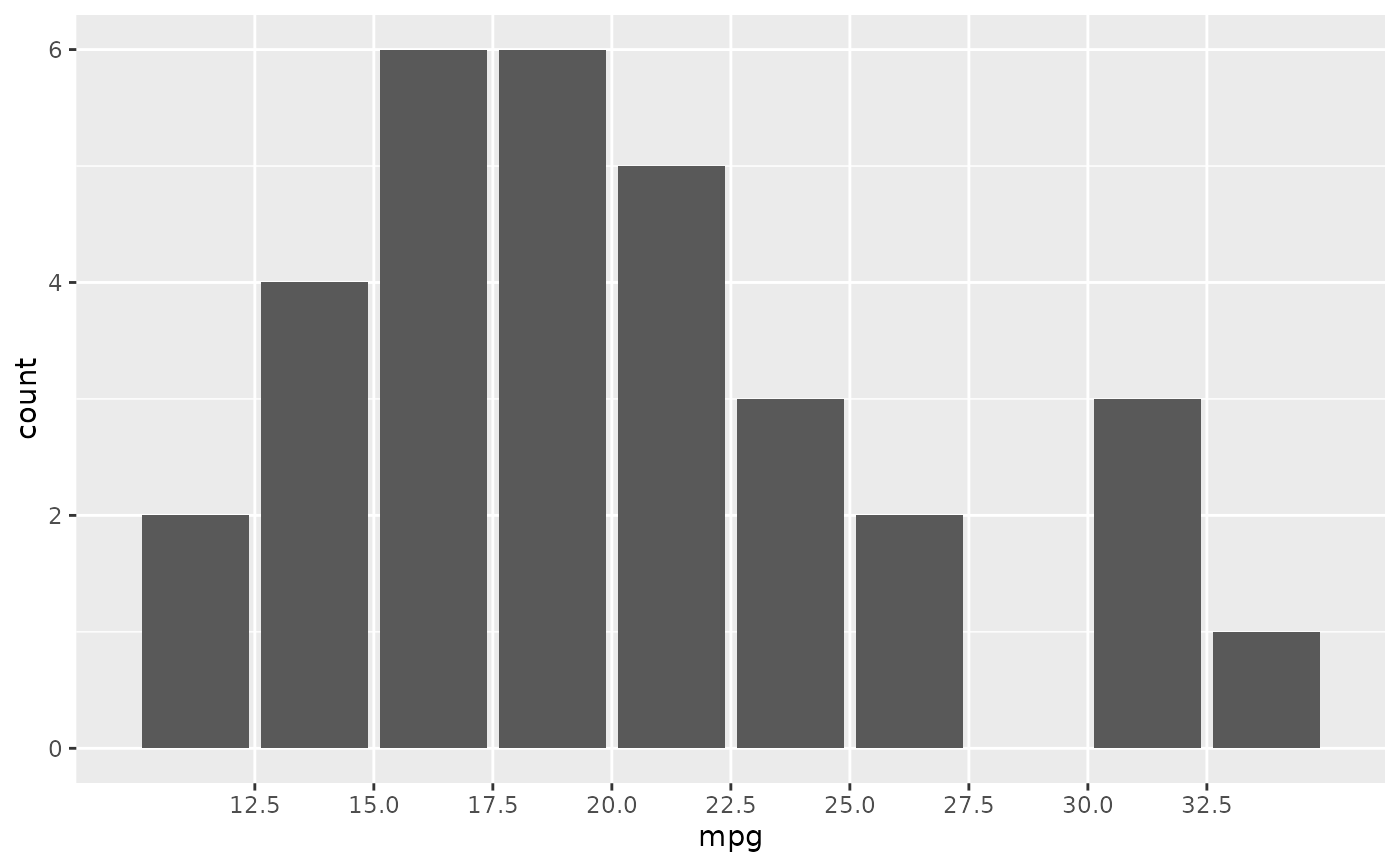scale_x_binned() and scale_y_binned() are scales that discretize continuous position data. You can use these scales to transform continuous inputs before using it with a geom that requires discrete positions. An example is using scale_x_binned() with geom_bar() to create a histogram.

## Usage

scale_x_binned(
name = waiver(),
n.breaks = 10,
nice.breaks = TRUE,
breaks = waiver(),
labels = waiver(),
limits = NULL,
expand = waiver(),
oob = squish,
na.value = NA_real_,
right = TRUE,
show.limits = FALSE,
trans = "identity",
guide = waiver(),
position = "bottom"
)

scale_y_binned(
name = waiver(),
n.breaks = 10,
nice.breaks = TRUE,
breaks = waiver(),
labels = waiver(),
limits = NULL,
expand = waiver(),
oob = squish,
na.value = NA_real_,
right = TRUE,
show.limits = FALSE,
trans = "identity",
guide = waiver(),
position = "left"
)

## Arguments

name

The name of the scale. Used as the axis or legend title. If waiver(), the default, the name of the scale is taken from the first mapping used for that aesthetic. If NULL, the legend title will be omitted.

n.breaks

The number of break points to create if breaks are not given directly.

nice.breaks

Logical. Should breaks be attempted placed at nice values instead of exactly evenly spaced between the limits. If TRUE (default) the scale will ask the transformation object to create breaks, and this may result in a different number of breaks than requested. Ignored if breaks are given explicitly.

breaks

One of:

• NULL for no breaks

• waiver() for the default breaks computed by the transformation object

• A numeric vector of positions

• A function that takes the limits as input and returns breaks as output (e.g., a function returned by scales::extended_breaks()). Also accepts rlang lambda function notation.

labels

One of:

• NULL for no labels

• waiver() for the default labels computed by the transformation object

• A character vector giving labels (must be same length as breaks)

• An expression vector (must be the same length as breaks). See ?plotmath for details.

• A function that takes the breaks as input and returns labels as output. Also accepts rlang lambda function notation.

limits

One of:

• NULL to use the default scale range

• A numeric vector of length two providing limits of the scale. Use NA to refer to the existing minimum or maximum

• A function that accepts the existing (automatic) limits and returns new limits. Also accepts rlang lambda function notation. Note that setting limits on positional scales will remove data outside of the limits. If the purpose is to zoom, use the limit argument in the coordinate system (see coord_cartesian()).

expand

For position scales, a vector of range expansion constants used to add some padding around the data to ensure that they are placed some distance away from the axes. Use the convenience function expansion() to generate the values for the expand argument. The defaults are to expand the scale by 5% on each side for continuous variables, and by 0.6 units on each side for discrete variables.

oob

One of:

• Function that handles limits outside of the scale limits (out of bounds). Also accepts rlang lambda function notation.

• The default (scales::censor()) replaces out of bounds values with NA.

• scales::squish() for squishing out of bounds values into range.

• scales::squish_infinite() for squishing infinite values into range.

na.value

Missing values will be replaced with this value.

right

Should the intervals be closed on the right (TRUE, default) or should the intervals be closed on the left (FALSE)? 'Closed on the right' means that values at break positions are part of the lower bin (open on the left), whereas they are part of the upper bin when intervals are closed on the left (open on the right).

show.limits

should the limits of the scale appear as ticks

trans

For continuous scales, the name of a transformation object or the object itself. Built-in transformations include "asn", "atanh", "boxcox", "date", "exp", "hms", "identity", "log", "log10", "log1p", "log2", "logit", "modulus", "probability", "probit", "pseudo_log", "reciprocal", "reverse", "sqrt" and "time".

A transformation object bundles together a transform, its inverse, and methods for generating breaks and labels. Transformation objects are defined in the scales package, and are called <name>_trans (e.g., scales::boxcox_trans()). You can create your own transformation with scales::trans_new().

guide

A function used to create a guide or its name. See guides() for more information.

position

For position scales, The position of the axis. left or right for y axes, top or bottom for x axes.

Other position scales: scale_x_continuous(), scale_x_date(), scale_x_discrete()
# Create a histogram by binning the x-axis